Reach Us+441474556909
Fiber Orientation and Aspect Ratio Calculation Using Image Analysis | OMICS International
Journal of Textile Science & Engineering
All submissions of the EM system will be redirected to Online Manuscript Submission System. Authors are requested to submit articles directly to Online Manuscript Submission System of respective journal.

# Fiber Orientation and Aspect Ratio Calculation Using Image Analysis

Akio Sakaguchi* and Hirokazu Kimura

Department of Advanced Textile Engineering, Faculty of Textile Science and Technology, Shinshu University, 3-15-1 Tokida, Ueda, Nagano 386-8567, Japan

*Corresponding Author:
Sakaguchi A
Department of Advanced Textile Engineering
Faculty of Textile Science and Technology
Shinshu University, 3-15-1 Tokida
Ueda, Nagano 386-8567, Japan
Tel: 81-268-21-5387
E-mail: [email protected]

Received Date: January 26, 2015; Accepted Date: February 09, 2015; Published Date: March 20, 2015

Citation: Sakaguchi A, Kimura H (2015) Fiber Orientation and Aspect Ratio Calculation Using Image Analysis. J Textile Sci Eng 5:187. doi: 10.4172/2165-8064.1000187

Copyright: © 2015 Sakaguchi A, et al. This is an open-access article distributed under the terms of the Creative Commons Attribution License, which permits unrestricted use, distribution, and reproduction in any medium, provided the original author and source are credited.

Visit for more related articles at Journal of Textile Science & Engineering

#### Abstract

As a preliminary study of fiber figure evaluation, the methodology of fiber orientation and aspect ratio calculation, especially that used in card webs, is discussed. To determine the fiber direction, the maximum variance method is introduced. In this method, we can derive the fiber’s main direction, which satisfies the criteria of maximum length and minimum width. Moreover, an experimental technique using image analysis is detailed. The results are consistent with the published studies. Thus, this method is viable and has a reliable theoretical background.

#### Keywords

Card web; Maximum variance method; Fiber orientation; Aspect ratio

#### Introduction

Carding is an important stage in textile processing for the manufacturing of spun yarns, nonwovens, and so on, from staple fibers. The performances and/or properties of the final products are affected by the fiber’s condition in card webs processed in the carding stage. For example, in spun yarn manufacturing, it is desirable for fibers in card webs to be parallel in machine direction to avoid floating fibers during roller drafting. It is important factor in improving evenness and mechanical performances of spun yarns. Hence, many studies have investigated the condition of fibers in card webs [1-3]. As shown in detailed study reported recently by Das et al. , this topic is still considered to be important in the present day. In this study, we discuss the calculation of fiber direction and aspect ratio using image analysis.

Fiber orientation distribution is an important function for card webs. First, we need to measure each fiber’s direction. There are several methods to determine a fiber’s direction. For example, the maximum distance length method has been used since the early decades . In this method, we select two points on the fiber such that the pair of points has the maximum distance length. Then, the direction of the line segment linking points is defined as the fiber’s direction. As another approach, Miao and Glassey have proposed the minimumwidth rectangle technique . They formed the narrowest rectangle to cover a fiber. The direction perpendicular to the narrower side of the rectangle is selected as the fiber’s direction.

Although these two intuitive methods are quite different, they have the same objective, i.e. to determine the direction of the maximum length or minimum width. However, it is unclear whether these techniques generate results consistent with each other. In this study, we propose the maximum variance method, which satisfies the criteria of maximum length and minimum width, theoretically. Then, we define the aspect ratio of a fiber using the obtained variances. Finally, this method is applied to experimental data.

#### Theoretical Discussion

Main direction

Figure 1 shows an example fiber image. In the figure, we have drawn two lines as candidates for the fiber’s direction. The points on the fiber are projected perpendicularly to the lines. Then, the variance of the projected point distribution is calculated. When the fiber and line direction coincide, the variance becomes large. By changing the line’s direction, we can find a line corresponding to the maximum variance. Now, we define the main direction of fiber, with the line’s direction corresponding to the maximum variance. Simultaneously, the direction perpendicular to the main direction corresponds to the minimum variance. The brief proof is described below.

Figure 1: Example of fiber image applied to the maximum variance method (Unit: μm).

We suppose that the coordinate origin O is placed at the center of gravity of the fiber. As shown in Figure 2, a point on the fiber is denoted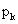, the distance from O tois denoted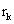, and the directional angle ofis denoted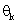. In this case, the coordinate values ofare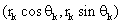. Therefore, the variances along the x- and y-axes are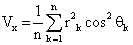and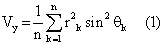respectively. The total variance is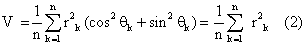Now, we rotate the coordinate axes clockwise by an angle of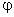and projectonto the rotated axes. The coordinate values on the rotated axes are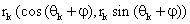.. Thus, the variances of both axes are as follows: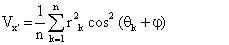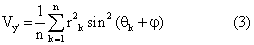The total variance is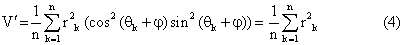That is, the total variance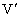is identical to that in Equation (2) and independent of. Hence, if we findcorresponding to maximum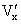., it results in minimum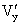, automatically. Consequently, in this method, we can simultaneously select the direction satisfying the criteria of maximum length and minimum width. Hence, this method is considered to be more advantageous in comparison with the reported intuitive techniques due to abovementioned theoretical background.

Aspect ratio

In this study, the aspect ratio of a fiber is defined as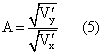Whereandare the maximized and minimized variances determined in Equation (3), respectively. In this case, to adjust the dimension of the parameters, square roots of the variances are used to define the aspect ratio. The aspect ratio takes a value from zero to one.

#### Experimental Details

Materials

We used polyester fibers (fiber length=38 mm, fiber fineness=1.4 dtex) as the raw material for our experiment. We dyed some of fibers to use as tracer fibers. Prior to carding, we mixed the dyed fiber with undyed fiber, and the content of dyed fiber was 0.1%.

Card

A flat card of the Shirley miniature spinning plant (Platt Bros. Ltd., England) was employed to process the prepared fibers. To mix the dyed fibers uniformly, the process was repeated twice.

Sampling

Referring to Miao and Glassey’s work, we sampled the carded webs in the following manner. We placed a sheet of A4 white paper on the collecting drum of the card as a web reinforcement. Then, we operated the card, and a thin sheet of web was produced on the paper. Next, we stopped the card and covered the web on the reinforcement paper with another sheet of paper. Then, we carefully removed the layer of the paper and web. Subsequently, we created a novel contrivance. We carefully removed the covering paper, and then sandwiched the sheet of web and the reinforcement paper with two sheets of transparent polyester film. Thereafter, we sealed them by heat using a laminator (Easy laminator KLA4, Iris Ohyama, Japan). The fibers in the web were fixed between the films, and we could observe the dyed fibers through the transparent film. As the laminated sheets were very easy to handle, we were able to use an image scanner (CanoScan LiDE 210, Canon, Japan) to capture the fibers’ images. We selected 4800 dpi and 8-bit gray scale mode of the scanner.

Image processing

Scanned images of dyed fibers were processed using an image processing software (WinTopo, SoftSoft Ltd., UK) and the fiber figure data were obtained. Using our own software programmed with Scilab (Scilab Enterprises, France), the length of each fiber was calculated. Some of the dyed fibers were partially overlaid by other fibers; thus, the calculated length of such fibers was much shorter than the original fiber length. In addition, fibers may fragment into short lint during carding. Hence, we excluded these 9 datasets from original 117 datasets and applied the following analysis to remaining 98 datasets. Thereafter, we resampled the fiber data into an equal-interval polyline with 3000 vertices. We related the vertices with the points on the fiber (Figure 1). Finally, the solver feature of Microsoft Excel (Microsoft, USA) was employed to determine the main direction of each fiber using the abovementioned method. We defined the fiber orientation angle as the angle deviation of the fiber direction from the machine direction. The obtained orientation and aspect ratio data were plotted in graphs and analyzed.

#### Results and Discussion

Captured images

Figure 3 shows example images of dyed fibers in card webs. In the figure, the machine direction is vertical. The arrows in the figure indicate the main directions derived using the maximum variance method. As shown in the figure, in many cases, fibers tend to be stretched in the machine direction rather than in the transverse direction. Thus, the main directions of fibers tended to be near machine direction. Horizontal directions of fibers in card webs, right and left, of fiber are not problem, but the angle deviations from the machine direction is important and described in the next subsection.

Fiber orientation angle

Figure 4 shows the fiber orientation distribution in card webs. In the figure, zero degrees means that the main direction of the fiber exactly matches the machine direction. Most fibers show a direction deviation smaller than 30 degrees. Despite the differences in the definition of the fiber direction among researchers, the trend of this result is consistent with published studies [3,5]. In the maximum distance method, only two points on the fiber play roles in determination of the fiber’s direction; hence, the resultant direction might be influenced by the movement of these points. Conversely, as 3000 points participate in the determination of fiber direction in our method, we can expect the obtained results to be robust.

Aspect ratio

Figure 5 shows the aspect ratio distribution of fibers in card webs. In the figure, a completely straight fiber has an aspect ratio of zero. However, we could not observe aspect ratios smaller than 0.1. In many cases, the aspect ratios are around 0.3, and mostly trailing-, leading-, or both-end-hooked fibers are observed, consistent with published studies . Because textile fibers are flexible material, tension is required to stretch fibers. Hence, these results were obtained. Similar results were obtained from the minimum-width rectangle technique . In their method, two points corresponding to minimum width and two points corresponding to maximum length on the fiber governed the results, and other points were ignored. Contrary, our method determine aspect ratios by the state of whole fiber; hence, it is effective.

#### Conclusion

To determine the direction of fibers in card webs, the maximum variance method is introduced. Using this method, the fiber direction is determined that satisfies maximum length and minimum width. Moreover, the aspect ratio of the fiber is defined in terms of the obtained maximum and minimum variances. In the experiment, laminated sheets of card webs were prepared and the resulting sheets were analyzed using an image scanner. From the captured data, the main direction and aspect ratio of each fiber were calculated using the proposed method. The results were consistent with the reported studies. Thus, the method introduced in this study is useful for practical measuring and has a reliable theoretical background.

#### Acknowledgements

We thank Mr. Shinya Sasaki for his assistance in the experiment. The authors would like to thank Enago for the English language review.

#### References

Select your language of interest to view the total content in your interested language

### Article Usage

• Total views: 13313
• [From(publication date):
February-2015 - Aug 18, 2019]
• Breakdown by view type
• HTML page views : 9436
• PDF downloads : 3877

## Post your commentCan't read the image? click here to refresh
###### Peer Reviewed Journals

Make the best use of Scientific Research and information from our 700 + peer reviewed, Open Access Journals

International Conferences 2019-20

Meet Inspiring Speakers and Experts at our 3000+ Global Annual Meetings

Top